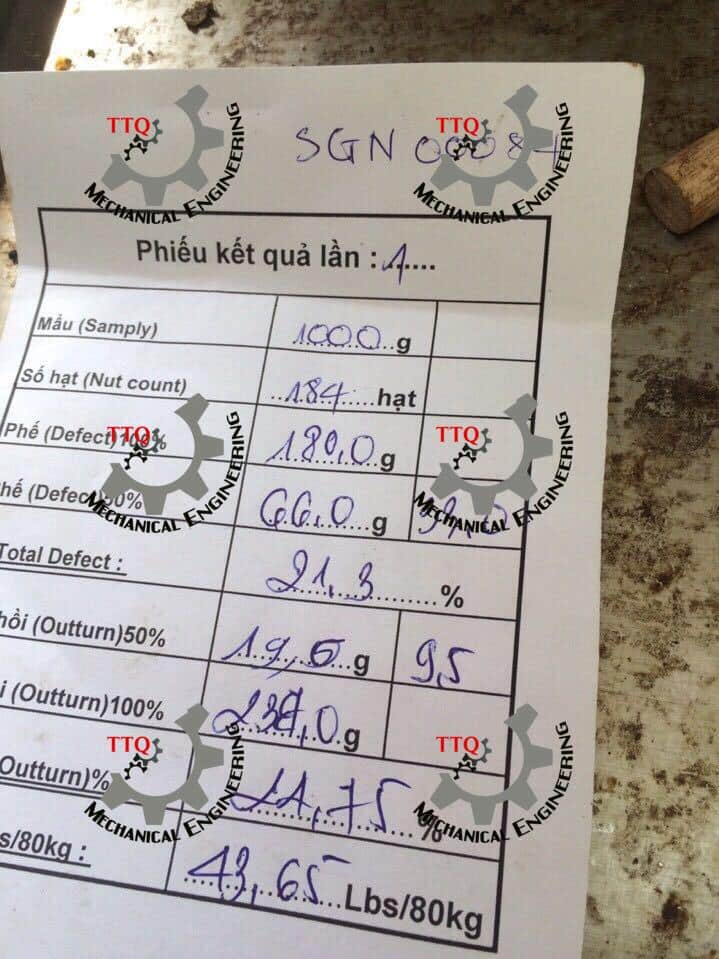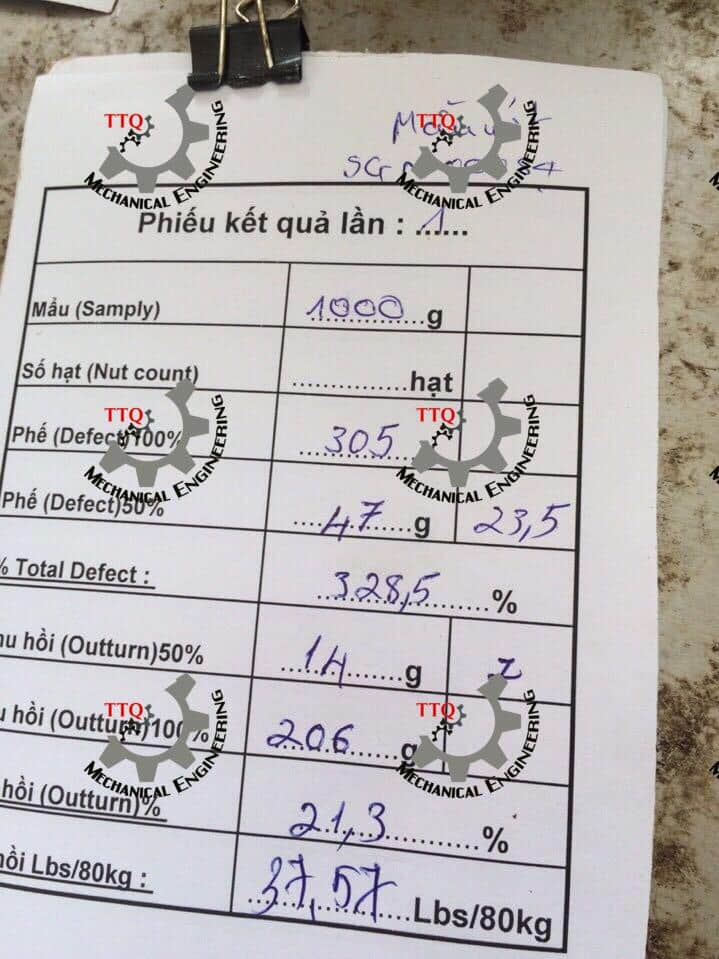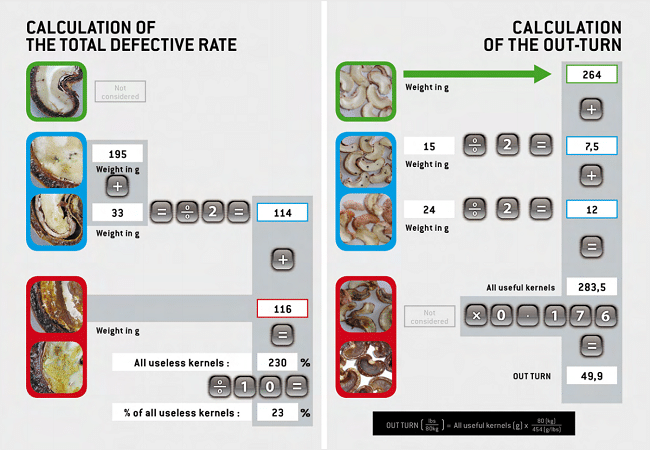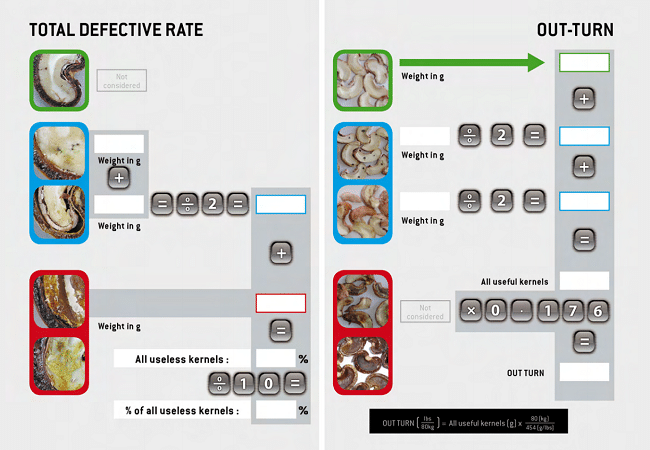# Cashew Outturn Calculation Formula | Cashew Outturn Ratio KOR

The Kernel Out-Turn is an most important parameter for the Cashew processor to control the nuts quality. The nut prices are determined according to the nuts quality. So its very important to understand the Cashew Outturn Calculation Formula.Understanding cashew new quality## Raw Cashew Nuts Outturn

Cashew processing is the process of removing the shell and kernel from the cashew nut. A sample cashew outturn calculation formula is done by multiplying the number of kilos of raw cashews by 0.75 to get the number of kilos of shelled cashews.
The formula for calculating cashew outturn is as follows:
Cashew Outturn = Number of kgs x 0.75
But this is just a rough estimate, it gives no details of actual outturn ratio nor the quality of the nuts. We will present here the physical criteria used by major cashew processors to access the quality of the Raw Cashew Nuts. These guidelines are from comcashew.com and will help you in quality control of raw cashew nuts.
Main Criteria used are:
– Out Turn (Kernel Output Ratio) also called as KOR
– Moisture
– Nut Count
– Total Defective Nuts

### Out Turn (Kernel Output Ratio) also called as KOR

Out-turn means the amount of usable kernels after de-shelling the nuts. It is expressed in lbs quantity, which just means the weight of useful kernels weighed in pounds in one 80 Kg Jute Bag of RCN (Raw Cashew Nut).
Out-turn can also be called KOR (Kernel Output Ratio) or kernels output.
1 Kg = 2.2 lbs
The Out-Turn is an most important parameter for the Cashew processor to control the nuts quality. The nut prices are determined according to the nuts quality.### The Defective Cashew Nut Rate

The defective cashew nuts rate gives the quantity of the defective nuts of the same: premature kernels, spotted kernels (blue category) + Stunted Kernels, Void Nuts, Moth eaten kernels, Mouldy kernels, Brown Kernels.
To determine this rate, we weight the kernels and the of these two categories. It is expressed in percentage. In general, a sample with more than 24% of defective nuts is rejected. This is faster and easier to calculate the defective rate than the out-turn, therefore it is used to have a first insight of the batch quality.

### The Nut Count

This is the number of nuts per kilogram, it is expressed in nuts/kg. In practice, it fluctuates between 150 and 240 nuts/kg. The smaller is the number, the bigger are the nuts.
Combined with the out-turn, the Nut Count gives information about the kernels size that we might get after the shelling.### The Moisture Rate

This is important element concerning the nuts storage. It is expressed in Percentage. It should be under control from harvesting to shelling. This is better to keep this rate under 10% after the drying. Over 10% nuts are likely to be mouldy, however if the rate is too low (under 6%) Kernels become dry and loose weight.
Moisture rate has to be between 7 and 10%## Making the Sample for Cashew Outturn Calculation

Small lots of nuts are taken randomly from different bags and are mixed together to make a Main Sample. This is further divided into 4 Parts of more of less equal parts called as quarter. So we have now 4 Quarters: two to two opposite.
Therefore:
-First Quarter is opposite to the third quarter
-Second quarter is opposite to the fourth quarter
Each sample has to come from 2 opposite quarters. For this, we take small amounts more or less equal of nuts in the opposite quarters, we mix them in a bucket.
Thus, we get:
Sample 1 from first Quarter and the third quarter
Sample 2 from the second quarter and the fourth quarter
Each sample, is weighed to get about 1 Kg of nuts. Let “W1” be the weight of a sample. It can fluctuate between 998kg and 1002kg
After weighing of the sample, we can calculate how many nuts are in the sample. This is “N”
Each nut (shell and kernel) of each sample is cut through with the help of Scissors, thus we get 2 halves with or without flaws. The split nuts are classified according to their characteristics. Make sure that the halves of each nut are kept together and that the kernels stay with the shells.
Kernels will be classified into three categories:
– Wholesome Kernels (100% accepted)
– 50% rejected Kernels
– 100 Rejected Kernels
Good Kernels are weighed with their peel and let them be “W2”
Weigh the Kernels + Shells of premature and spotted, Let “W3” be the total weight of the nuts (Kernels+shells) 50% accepted.
Kernels (premature and spotted) are removed from the shells. All these Kernels are 50% accepted, mark them as “W4”
Defective kernels (mouldy, brown, motheaten, empty and stunted) is weighed with Shells. Let “W5” be this total weight of the Nuts (Shell + Kernels) 100% rejected.

## Calculation of the Nut Count

This is the number of nuts per kilogram. It is calculated by dividing the number of nuts in the sample by the weight of this sample.

W1: Weight of the sample

N: Number of the nuts counted in one sample

Nut Count = N/W1

## Calculation of the total defective nut rate

W1: Total weight of the nuts sample

W5: Weight of the 100% rejected nuts

W3: Weight of the 50% rejected nuts

The defective rate is: (W3+W5)/W1 x 100

## Calculation of the Cashew Kernels productivity, quality and of the Out-Turn

W1: Total weight of the nuts sample

W4: Total weight of Kernels + Peels of 50% rejected Nuts

W2: Total Weight of Kernels + Peels of the good Kernels.

% of useful Kernels = (W2+(W4/2))/W1 x 100

For the processors, the processing of cashew nuts are conducted on base of 80 Kg-nuts bag and of a weight of kernels expressed in pounds (lb)

Out-turn = % of useful kernels/((100 x 80 x (1/0.45349))Calculating Outturn of Cashew Nuts

## HS Code for Raw Cashew Nut

HS Code for Cashew nuts:
08013100 - In shell
08013200 - Shelled

## Please fill this Contact form for Cashew Nut Roasting Machine details & price.

#### Phone

+84 97676 5127